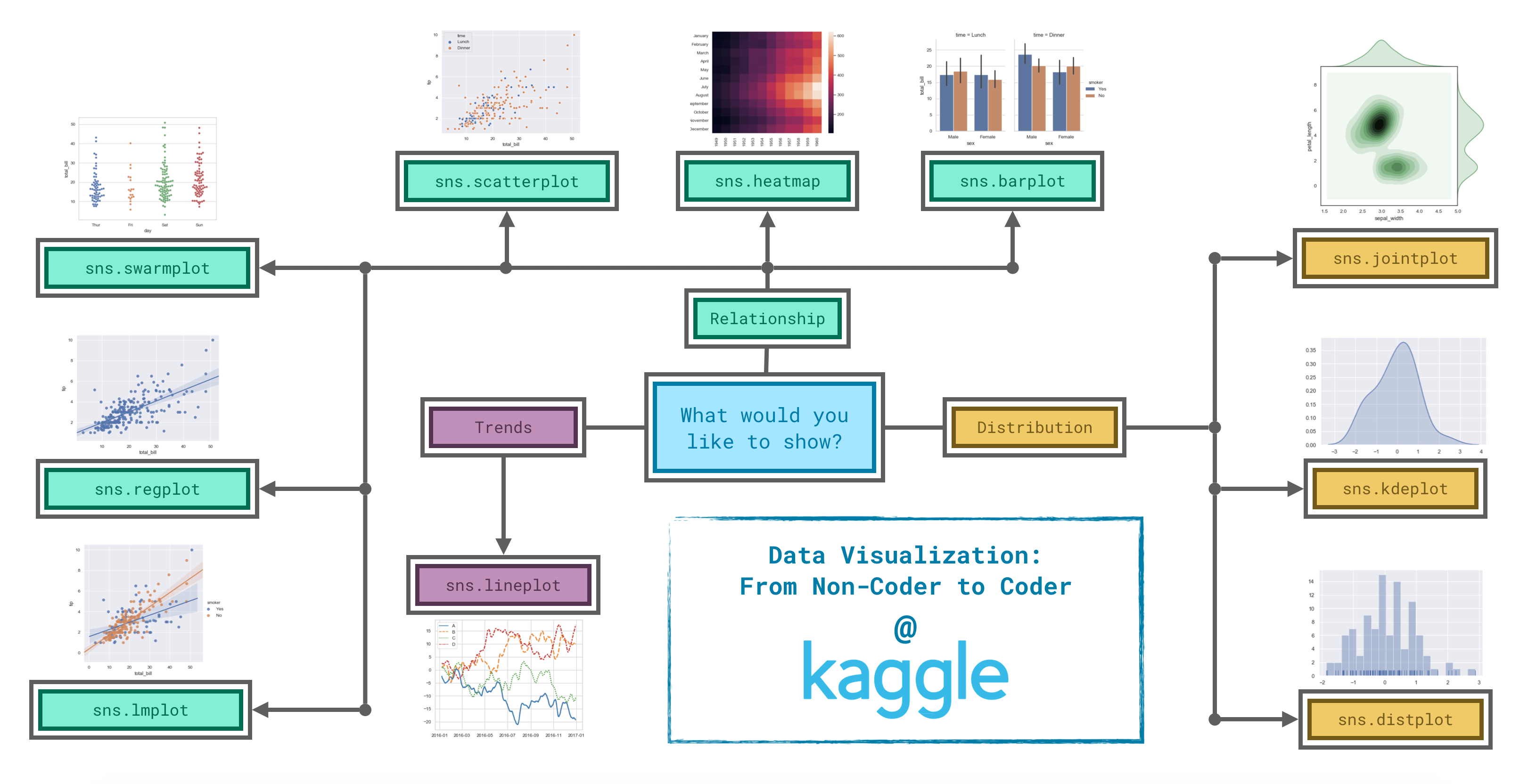Python Plotly

## Seaborn• Trends - A trend is defined as a pattern of change.
• sns.lineplot - Line charts are best to show trends over a period of time, and multiple lines can be used to show trends in more than one group.
• Relationship - There are many different chart types that you can use to understand relationships between variables in your data.
• sns.barplot - Bar charts are useful for comparing quantities corresponding to different groups.
• sns.heatmap - Heatmaps can be used to find color-coded patterns in tables of numbers.
• sns.scatterplot - Scatter plots show the relationship between two continuous variables; if color-coded, we can also show the relationship with a third categorical variable.
• sns.regplot - Including a regression line in the scatter plot makes it easier to see any linear relationship between two variables.
• sns.lmplot - This command is useful for drawing multiple regression lines, if the scatter plot contains multiple, color-coded groups.
• sns.swarmplot - Categorical scatter plots show the relationship between a continuous variable and a categorical variable.
• Distribution - We visualize distributions to show the possible values that we can expect to see in a variable, along with how likely they are.
• sns.distplot - Histograms show the distribution of a single numerical variable.
• sns.kdeplot - KDE plots (or 2D KDE plots) show an estimated, smooth distribution of a single numerical variable (or two numerical variables).
• sns.jointplot - This command is useful for simultaneously displaying a 2D KDE plot with the corresponding KDE plots for each individual variable.

### Histograms and Density Plots

#### Histograms

• x=: chooses the column we’d like to plot.
• kde=False: Whether to plot a gaussian kernel density estimate..

#### Density Plots (KDE)

• fill: If True, fill in the area under univariate density curves or between bivariate contours. If None, the default depends on multiple.

#### 2D KDE Plots

Draw a plot of two variables with bivariate and univariate graphs.

• kind: kind : { “scatter” | “kde” | “hist” | “hex” | “reg” | “resid” }
• Kind of plot to draw. See the examples for references to the underlying functions.

#### Color-coded plots (multiple histograms)

• label= is used to set the values in the legend.

### Choosing Plot Types and Custom Styles

Seaborn has five different themes: (1)”darkgrid“, (2)”whitegrid“, (3)”dark“, (4)”white“, and (5)”ticks“.

### Multi-plot Grids

Building structured multi-plot grids